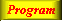Lecture 4 - Page 24 : 34
 Functional Programming in SchemeHigher-order Functions * Introduction to higher-order functions Higher-order functions Some simple and general higher-order functions Linear search in lists Generation of list selectors * Mapping and filtering Classical higher-order functions: Overview Mapping The mapping function Examples of mapping Filtering The filtering function Examples of filtering * Reduction and zipping Reduction The reduction functions Accumulation Zipping The zipping function * Currying The idea of currying Currying in Scheme Examples of currying Ad hoc currying in Scheme (1) Ad hoc currying in Scheme (2) * Web related higher-order functions HTML mirror generation HTML mirror usage examples Making tables with the real mirror Tables with higher-order functions HTML element modifications The function simple-html-table The XHTML mirror in LAML Generation of a leq predicate from enumeration
 Ad hoc currying in Scheme (1)
 It is possible to achieve 'the currying effect' by generalizing functions, which requires two or more parameters, to only require a single parameter
 Motivation:
 Expression Value `(map li (list "one" "two" "three"))` ```("
• one
• " "
• two
• " "
• three
• ")``` `(define li-mapper (map li))` map: expects at least 2 arguments, given 1 ```(define li-mapper ((curry2 map) li)) (li-mapper (list "one" "two" "three"))``` ```("
• one
• " "
• two
• " "
• three
• ")```

A legal mapping and an impossible attempt to curry the mapping function. The last example shows an application of curry2 to achieve the wanted effect, but as it appears, the solution is not very elegant.The function curry-generalized.

This is a higher-order function which generalizes the function passed as parameter to curry-generalized. The generalization provides for just passing a single parameter to f, in the vein of currying.